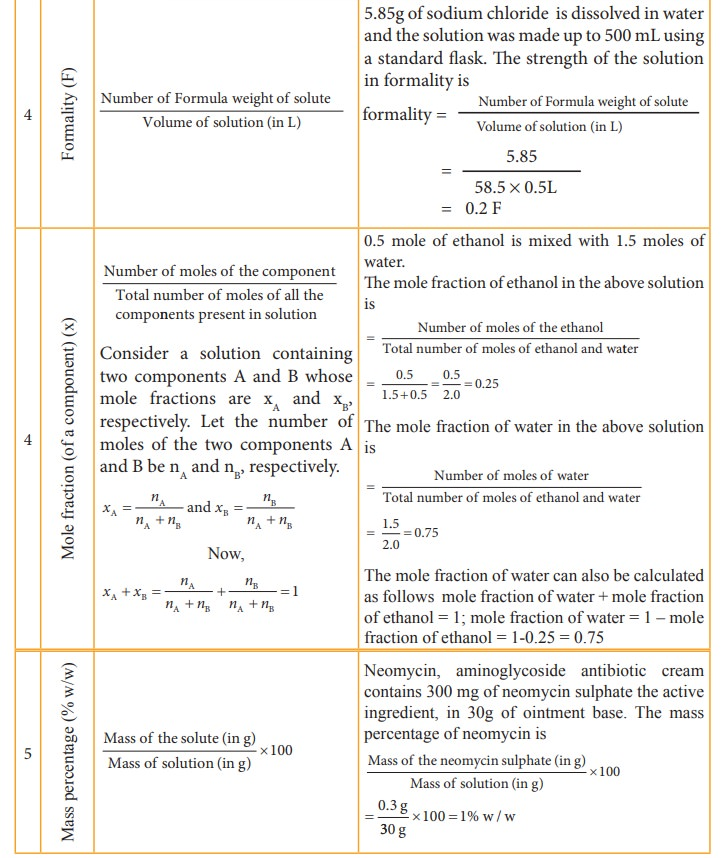Home | | Chemistry 11th std | Expressing concentration of solutions

# Expressing concentration of solutions

The concentration of a solution is expressed in different units. The choice of unit depends on the type of measurement applied.

Expressing concentration of solutions

In our life we have come across many solutions of varying strengths or concentrations such as mouthwash, antiseptic solutions, household disinfectants etc... Have you ever noticed the concentration of the ingredients present in those solutions? For example, chlorhexidine mouthwash solution contains 0.2 % (w/v) chlorhexidine gluconate; The concentration of the commercially available hydrogen peroxide is 3% (w/v). Similarly, other terms such as ppm (TDS of water), molar and normal (laboratory reagents) are used to express the concentration of the solution. The concentration of a solution gives the amount of solute present in a given quantity of solvent. As we have seen, there are different ways of expressing the concentration of a solutions. Let us learn the different concentration terms and to prepare a solution of a specific concentration.

### Table 9.2 Different concentration units and their illustrationsThe concentration of a solution is expressed in different units. The choice of unit depends on the type of measurement applied. For example,in complexometric titrations involving EDTA, the reaction between EDTA and the metal ions takes place in the 1:1 mole ratio and hence molar solutions are used in this titrations. In the redox and neutralisation titrations we use normality. The mole fraction is used to calculate the partial pressure of gases and the vapour pressure of solutions. The percentage units are used to express the active ingredients present in therapeutics, and the ppm is used to express the quantity of solutes present in small amounts in solutions.

## Standard solutions and working standards

A standard solution or a stock solution is a solution whose concentration is accurately known. A standard solution of required concentration can be prepared by dissolving a required amount of a solute, in a suitable amount of solvent. Its done by transforming a known amount of a solute to a standard flask of definite volume. A small amount of water is added to the flask and shaken well to dissolve the salt.. Then water is added to the flask to bring the solution level to the mark indicated at the top end of the flask. The flask is stoppered and shaken well to make concentration uniform.

At the time of experiment, the solution with required concentration is prepared by diluting the stock solution. This diluted solution is usually called working standard. A known volume of stock solution is transferred to a new container and brought to the calculated volume. The necessary volumes of the stock solution and final volume can be calculated using the following expression.

Cs Vs = Cw Vw (9.1)

Where the Cs &Vs are concentration and the volume of the stock solution and Cw & Vw are concentration and the volume of the working standard, respectively.## Advantages of using standard solutions:

1. The error due to weighing the solute can be minimised by using concentrated stock solution that requires large quantity of solute.

2. We can prepare working standards of different concentrations by diluting the stock solution, which is more efficient since consistency is maintained.

3. Some of the concentrated solutions are more stable and are less likely to support microbial growth than working standards used in the experiments.

## Example Problem

1. What volume of 4M HCl and 2M HCl should be mixed to get 500 mL of 2.5 M HCl?

Let the volume of 4M HCl required to prepare 500 mL of 2.5 MHCl = x mL

Therefore, the required volume of 2M HCl = (500 - x) mL

We know from the equation (9.1)

C1V1+ C2V2 =   C3V3

(4x)+2(500-x)       =       2.5 Ă— 500

4x+1000-2x         =       1250

2x  = 1250 - 1000

x    = 250/2

= 125 mL

Hence, volume of 4M HCl required = 125 mL

Volume of 2M HCl required = (500 - 125) mL= 375 mL

Tags : Chemistry , 11th Chemistry : UNIT 9 : Solutions
Study Material, Lecturing Notes, Assignment, Reference, Wiki description explanation, brief detail
11th Chemistry : UNIT 9 : Solutions : Expressing concentration of solutions | Chemistry